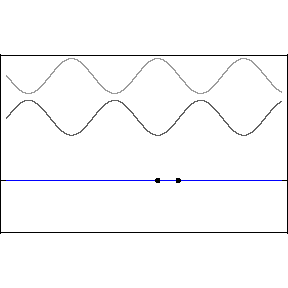# Lecture 12: Standing Waves; Wave Interference

## Wave Interference

• Interference is one of the most fundamental and important phenomena of the physics of waves.

• Intereference is what happens when you have more than one wave. It is how they add up
 Two identical waves Two waves, same frequency but different amplitude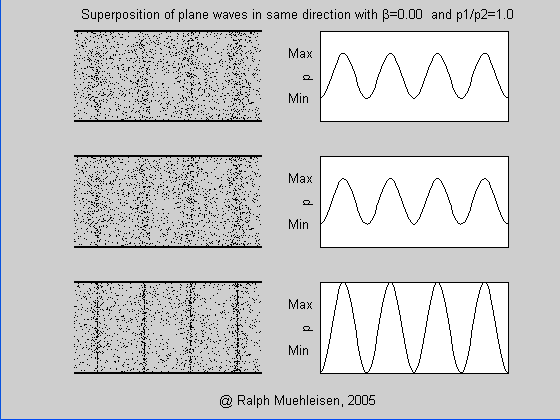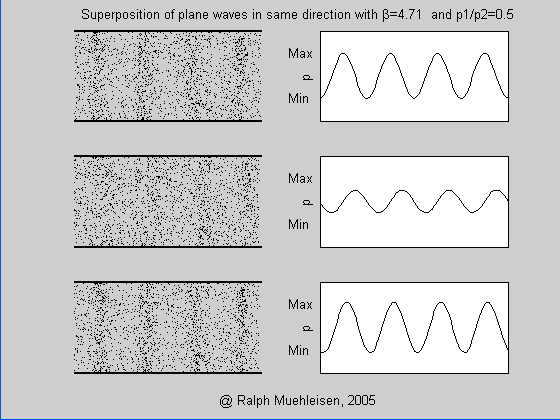Two identical waves but traveling in opposite direction Two waves, same frequency but different amplitude, traveling in opposite direction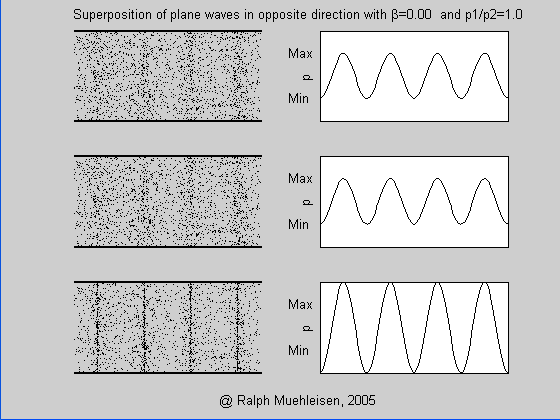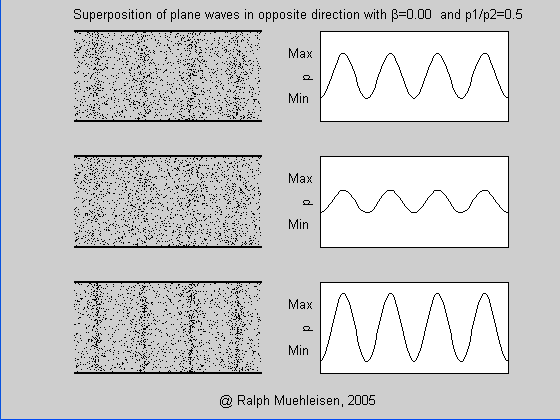• How to create multiple waves ? One way - reflect the wave of the boundary of the medium. Let us look at again at very useful applet in our textbook.
Next

## Principle of superposition: Wave functions simply add up

• If I have two waves, 1 and 2, together they are described by simple sum of their wave functions

y( x,t ) = y1( x,t ) + y2( x,t )

• Principle of superposition reflects linear (in y(x,t) ) nature of the wave equation
Next

## Standing Waves

• Especially important example of superposition is the effect of standing wave
• Standing wave is formed when two waves of the same amplitude and freguency, travelling with the same speed in opposite directions interfere (add up).
 Experiment Theory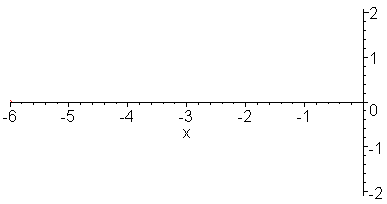• Wave function of the standing wave obtained by reflection at x=0 (inverted amplitude) is
y ( x,t ) = A cos( k x - ω t) - A cos( k x + ω t )
• or
y ( x,t ) = 2 A sin( k x ) sin ( ω t)
Next

## Properties of the Standing Waves

 Amplitude is twice Asw = 2 A Different places x experience different oscillations Some have no motion at all, sin( k x ) = 0 . These are called nodes . Nodes are positioned at x = ½ n λ Some experince maximum swing, sin( k x ) = 1 . The are called antinodes . Antinodes are positioned at x = ½ n λ + ¼ λ All points between two nodes move in phase There is no transport of energy over large distance